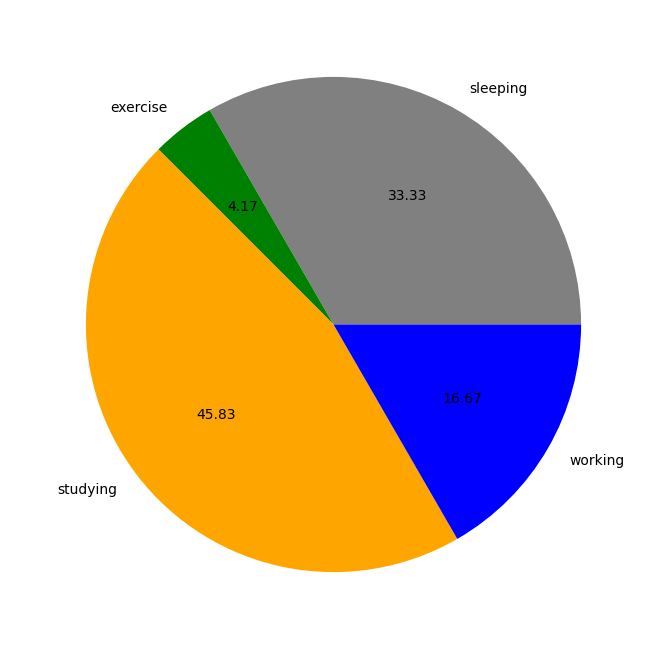# How to remove the label on the left side in matplotlib.pyplot pie charts?

MatplotlibPythonData Visualization

To remove the label on the left side in a matplotlib pie chart, we can take the following steps −

• Create lists of hours, activities, and colors.

• Plot a pie chart using pie() method.

• To hide the label on the left side in matplotlib, we can use plt.ylabel("") with ablank string.

## Example

import matplotlib.pyplot as plt
plt.rcParams["figure.figsize"] = [7.00, 3.50]
plt.rcParams["figure.autolayout"] = True
hours = [8, 1, 11, 4]
activities = ['sleeping', 'exercise', 'studying', 'working']
colors = ["grey", "green", "orange", "blue"]
plt.pie(hours, labels=activities, colors=colors, autopct="%.2f")
plt.ylabel("")
plt.show()

## Output